## Example Questions

### Example Question #94 : Trigonometry

Simplify: (sinΘ + cosΘ)2

cos2Θ -1

2sinΘcosΘ -1

1 + cos2Θ

1 + sin2Θ

None of the answers are correct

1 + sin2Θ

Explanation:

Using the foil method, multiply.  Simplify using the Pythagorean identity sin2Θ + cos2Θ = 1 and the double angle identity sin2Θ = 2sinΘcosΘ.

### Example Question #95 : Trigonometry

For the triangle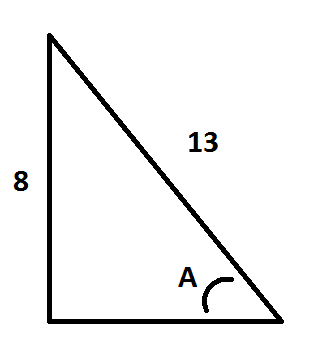, find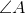in degrees to the nearest integer

Note: The triangle is not necessarily to scale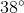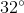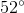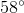Explanation:

To solve this equation, it is best to remember the mnemonic SOHCAHTOA which translates to Sin = Opposite / Hypotenuse, Cosine = Adjacent / Hypotenuse, and Tangent = Opposite / Adjacent. Looking at the problem statement, we are given the side opposite of the angle we are trying to find as well as the hypotenuse. Therefore, we will be using the SOH part of our mnemonic. Inserting our values, this becomes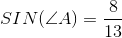. Then, we can write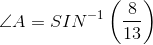. Solving this, we get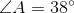### Example Question #96 : Trigonometry

A fifteen foot ladder is leaned up against a twelve foot building, reaching the top of the building. What is the angle made between the ladder and the ground? Round to the nearest hundredth of a degree.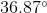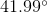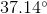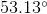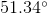Explanation:

You can draw your scenario using the following right triangle: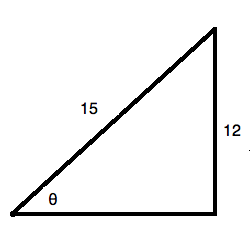Recall that the sine of an angle is equal to the ratio of the opposite side to the hypotenuse of the triangle. You can solve for the angle by using an inverse sine function: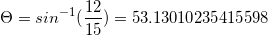or.

### Example Question #97 : Trigonometry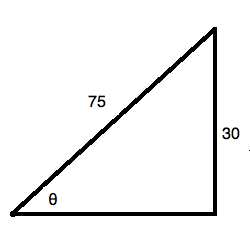What is the value of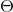in the right triangle above? Round to the nearest hundredth of a degree.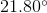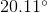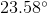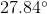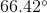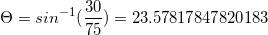or.